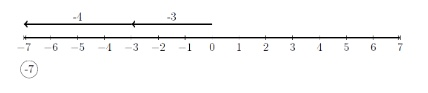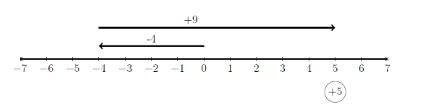# Applied Mathematics Study Guide for the WorkKeys

Page 1

## General Information

This test is one of the three required in the basic WorkKeys® exam, the other two being Graphic Literacy and Workplace Documents. Passing all three tests will entitle you to receive the National Career Readiness Certificate.

You will have 55 minutes to answer 34 multiple-choice questions, all of which are presented as “word problems.” For each, you’ll need to take the information given and create calculations to find the answer. You will not be penalized for guessing, so try to answer all questions on the test.

The questions encompass five levels of difficulty, from 3 through 7. Here is a brief description of the type of question you’ll find on each level:

• Level 3: Only simply-presented information that you need to solve the problem is included.

• Level 4: Information may not be presented in the order you need it and the question may include extra information that you don’t need. These are mostly only numeric calculations required.

• Level 5: These questions may require steps to solve and you may need to convert all numbers to one form (decimals/fractions, etc.) before solving. Some geometry questions are included.

• Level 6: Basically, this level of question can require you to do things like create more than one equation, do more complicated calculations, work with unit conversions, and deal with more complex geometry concepts.

• Level 7: Questions on this level may require you to sift through information that is not given in a clear-cut manner, but needs to be inferred. There also may be even more advanced geometry concepts assessed as well as basic statistics.

Note that questions on each level also assume you have the skills required on the previous level.

Also, you will have access to a list of basic formulas during this test and you may use a basic four-function calculator. Check with your test center to see if one will be provided online or which kind is approved for use during the test.

## Skills Needed for Level 3 Questions

Level 3 questions are the most basic you will face. If there are word problems, they will be easy to write as a math expression or equation. All the information you need will be given logically with no extra information to confuse things.

### Basic Mathematical Operations

Only one of the following operations will be needed to calculate answers to problems on this level. In other words, they will be one-step problems.

#### Adding Positive and Negative Numbers

If the two numbers have the same sign, simply add them together and give the answer the common sign of the two numbers you added. For example:

$(+7)+(+11)=+18$ $(-10)+(-19)=-29$

If the two numbers have different signs, assume both signs are positive, and subtract the smaller numeral from the larger one. (A more correct way to say this is “subtract the absolute values” of the two numbers.) Then give the answer the sign that the larger numeral originally had. For example:

$(-13)+(+9)=$ $13-9 =4$

$$13$$ is larger than $$9$$, so use its original sign ($$-$$) for the answer.
Answer: $$-4$$

$(+15)+(-8)=$ $15-8=7$

$$15$$ is larger than $$8$$, so use its original sign ($$+$$) for the answer.
Answer: $$7$$

If the method above is confusing, here is another way to look at adding, using a number line.

To add $$(+6)+(-10)$$ remember that on a number line the positive direction is to the right and the negative direction is to the left.
To show $$+6$$, start at zero and draw an arrow to the right six units long.
To show adding $$-10$$, start at the number six and draw an arrow ten units long to the left.
The second arrow always starts from where the first arrow ends.
The result is where the second arrow ends.Here is another example, this time adding numbers with the same sign:

$-4+-3=-7$#### Subtracting Positive and Negative Numbers

Subtraction can be handled by the rules of addition above. First, change the sign of the second number and add the two numbers using the rules of addition above. For example:

$(+7)-(+11)=$

Change the sign of the $$11$$ and add.

$(+7)+(-11)=$

The signs are now different, so the rule of addition says to ignore the original signs, subtract $$7$$ from $$11$$, and give the answer the sign of the larger number, which is ($$-$$).

$11-7=4$ $-4$

Another example:

$(-15)-(-18)=$

Change the sign of the $$18$$ and add.

$(-15)+(+18)=$

Now, it’s an addition problem and the signs are different, so the rule of addition says to ignore the signs, subtract $$15$$ from $$18$$ and give the answer the sign of the $$18$$, which is ($$+$$).

$18-15=3$

A good way to think of subtraction is this: Subtracting means the same as adding the opposite. If you’re subtracting $$8$$, that’s the same as adding a $$-8$$. If you’re subtracting $$-8$$, that’s the same as adding an $$8$$.

We can show this on a number line too.

$$(+5)-(+11)$$ is the same as $$(+5)+(-11)$$.$(+5)+(-11)=(-6)$

One more example:

$$(-4)-(-9)$$ is the same as $$(-4)+(+9)$$$(-4)+(+9)=(+5)$

#### Multiplying Only Positive Numbers

When multiplying only positive numbers, you won’t have to worry about whether the answer is positive or negative, but you do need to be able to multiply various types of numbers, large and small. If basic multiplication is hard for you, you can find many practice sites online to help you work on this skill.

#### Dividing Only Positive Numbers

Like basic multiplication, knowing how to do basic division calculations is important for this test. You’ll need to be able to handle division of all sorts of numbers, large and small. No, you don’t have to worry about the signs if all the numbers are positive, but you do need to be able to divide accurately. Seek additional practice on this if it gives you trouble.

#### Multiplying and Dividing Signed Numbers

Please keep in mind that, even though you will have access to a calculator on the test, knowing the multiplication tables really well will pay off in a better understanding of multiplication and division, as well as speeding up your problem solving.

Whether multiplying or dividing signed numbers, there is only one rule.

If the number of negative numbers is odd, the answer is negative. Examples:

$-3 \times 5 = -15$ $-2 \times -9=18$ $-4 \times 6 \times -2=48$ $-24 \div -6 = 4$ $33 \div -11=-3$ $-3 \times -4 \div -2 \times -7=42$

(Remember to work from left to right.)

For much more information about basic operations and other math stuff, be sure to check out our Math Basics Materials.

### Converting Number Types

Be able to convert among fractions, decimals, and percents.

#### Fraction to Decimal

Fractions can be converted to decimals by dividing the top number (numerator) by the bottom number (denominator).

Examples:

$\dfrac{3}{4}= 3 \div 4 = 0.75$ $\dfrac {5}{9}=5 \div 9 = 0.555\bar{5}$

The bar over the last $$5$$ shows that fives keep repeating forever.
Depending on the problem, the number $$0.555\bar{5}$$ may be rounded. For example:

$$0.556$$, $$0.56$$, or $$0.6$$

#### Decimal to Fraction

It’s good to remember a few simple decimal and fractional equivalents that come up often. For example:

$\dfrac{1}{4}=0.25$ $\dfrac{1}{2} = 0.5$ $\dfrac{3}{4}=0.75$ $\dfrac{1}{3} = 0.33\bar{3}$ $\dfrac{2}{3}=0.66\bar{6}$

For other decimals, you can convert by knowing decimal place values.

$$0.3$$ is the same as $$\dfrac{3}{10}$$

$$0.12$$ is the same as $$\dfrac{12}{100}$$.

Reduce it to $$\dfrac{3}{25}$$.

#### Decimal to Percentage

Converting decimals to percentages is a piece of cake. All you have to do is move the decimal two places to the right.

$0.45= 45\%$ $0.066=6.6\%$ $1.08=108\%$

### Converting Units

Many problems involve units, and very often, they come in forms that need to be converted to different forms: cents to dollars, seconds to hours, miles to feet, and so forth.

#### Money

Since it takes $$100$$ cents to make a dollar, it makes sense to say that one cent is a hundredth of a dollar. This is a lucky break if you need to change from one to the other, because all you need to do is move the decimal point two places. To get cents from dollars, the number must get larger, so move the decimal point two places to the right:

$$\1.38 = 138$$ ¢

To get dollars from cents, the number must get smaller, so move the decimal point two places to the left:

$$595$$ ¢ $$= \5.95$$

#### Time

Common time units are weeks, days, hours, minutes, and seconds. You definitely should know that:

$$1$$ week = $$7$$ days
$$1$$ day = $$24$$ hours
$$1$$ hour = $$60$$ minutes
$$1$$ minute = $$60$$ seconds

You can use the method below to convert from any of these units to any other unit. If you are converting to a unit to the right in the table, multiply as shown by each number you pass over as you go toward the unit you want.

In Level $$3$$, the problems will be only one step, say from days to hours or from minutes to seconds. Higher-level questions may require multiple steps, as shown in the examples below.

$\text{Weeks} \times 7 = \text{days} \times 24 = \text{hours} \times 60 = \text{minutes} \times 60 = \text{seconds}$

Example: To change $$2$$ days to minutes, multiply days by $$24$$ to get hours, and then multiply that by $$60$$ to get seconds.

$2 \times 24 \times 60 = 2880 \text { minutes}$

If you are converting to a unit to the left in the table, you need to do the opposite; divide by each number you pass over as you go toward the unit you want.

Example: To change $$10,800$$ seconds to hours, divide by $$60$$ to get minutes, and divide by $$60$$ to get hours.

$10,800 \div 60 \div 60 = 3 \text{ hours}$

### Making Change

Basic money handling is good to know for this test. It involves adding items to get a total amount due and calculating the amount of change the customer should get back.

Adding quantities of money is basically just adding numbers with decimals, although you do have to be careful that all units are expressed in the same form. That form is almost always going to be dollars, so if you wanted to add $$\7.88$$ and $$69$$ ¢, change the $$69$$ ¢ to $$\0.69$$ and add that..
$\begin{array}{r} &\7.88 \\ &+\0.69 \\ \hline &\8.57 \end{array}$
Determining the change you should get back when you buy something is a subtraction problem. Take the amount you gave the clerk and subtract the total purchase price. Let’s say you gave $$\40.00$$ for some groceries that cost $$\32.74$$. Your change back should be:
$\begin{array}{r} &\40.00 \\ &-\32.74 \\ \hline &\7.26 \end{array}$